Home > CCA2 > Chapter 11 > Lesson 11.3.1 > Problem11-91

11-91.
1. Multiply and simplify each expression. Homework Help ✎

1. (3 + 2i)(4 + i)

2. (2 + 3i)(2 − 3i)

3. (5 − 2i)(5 + 2i)

4. (a + bi)(abi)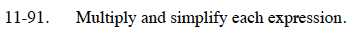Use an area model. Remember that i2 = −1.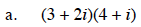12 + 3i + 8i + 2i2

10 + 11i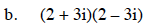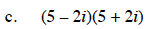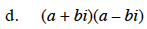a2 + b2